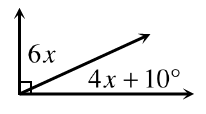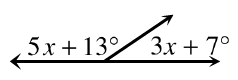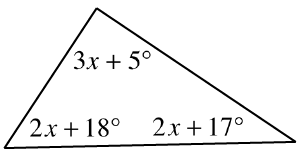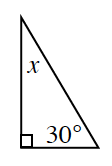### Home > CCAA > Chapter 9 Unit 10 > Lesson CC3: 9.2.3 > Problem9-96

9-96.

For each diagram below, solve for $x$. Explain what method you used for each problem.

1.The two angles are complementary, meaning they have a sum of $90°$.

1.The two angles are supplementary (have a sum of $180°$).

$x=20°$

1.According to the Angle Sum Theorem, the sum of the interior angles of a triangle is $180°$.

1.How did you solve part (c)? Use the same method.

$x + 30 + 90 = 180$

$x + 120 = 180$

$x=60°$